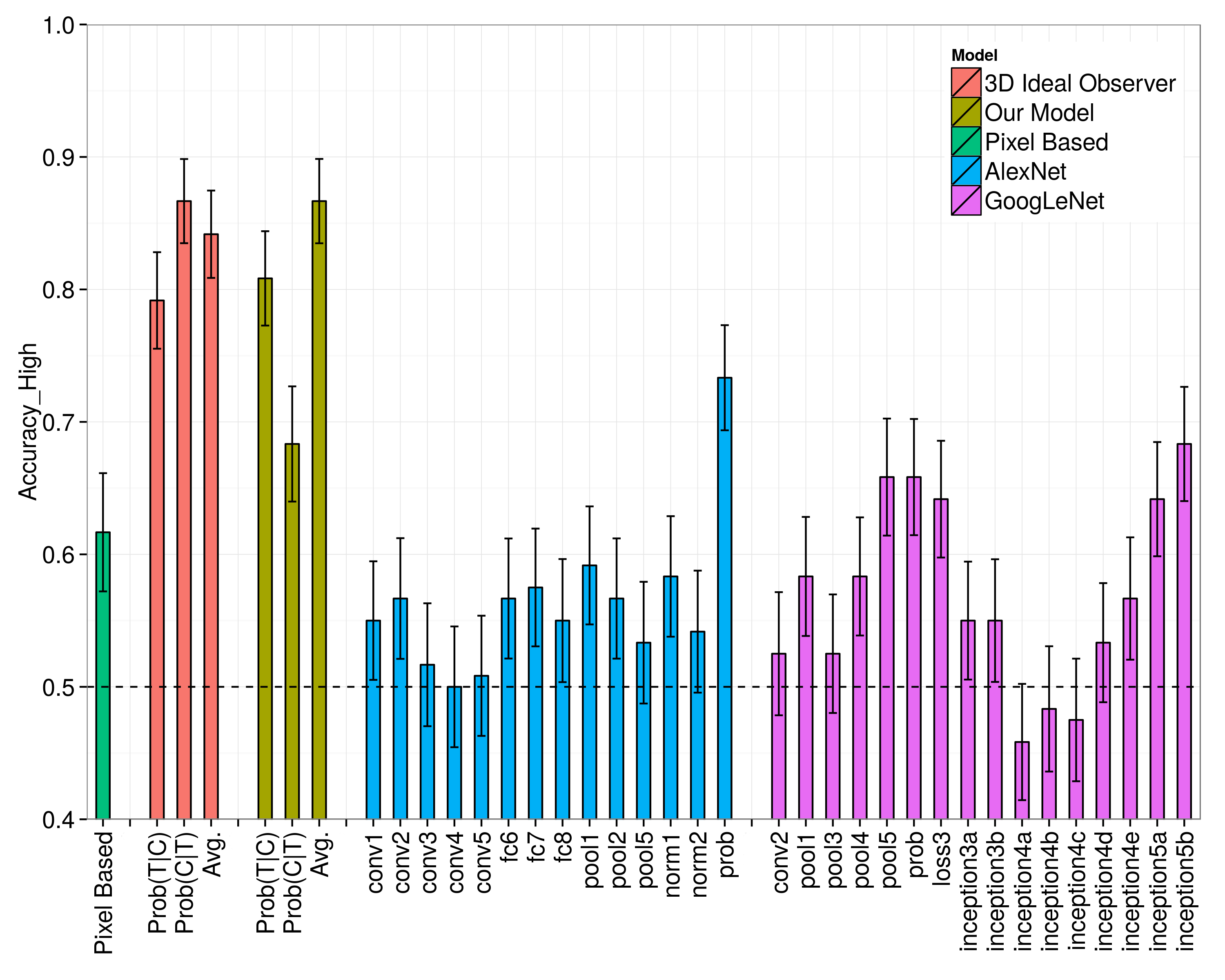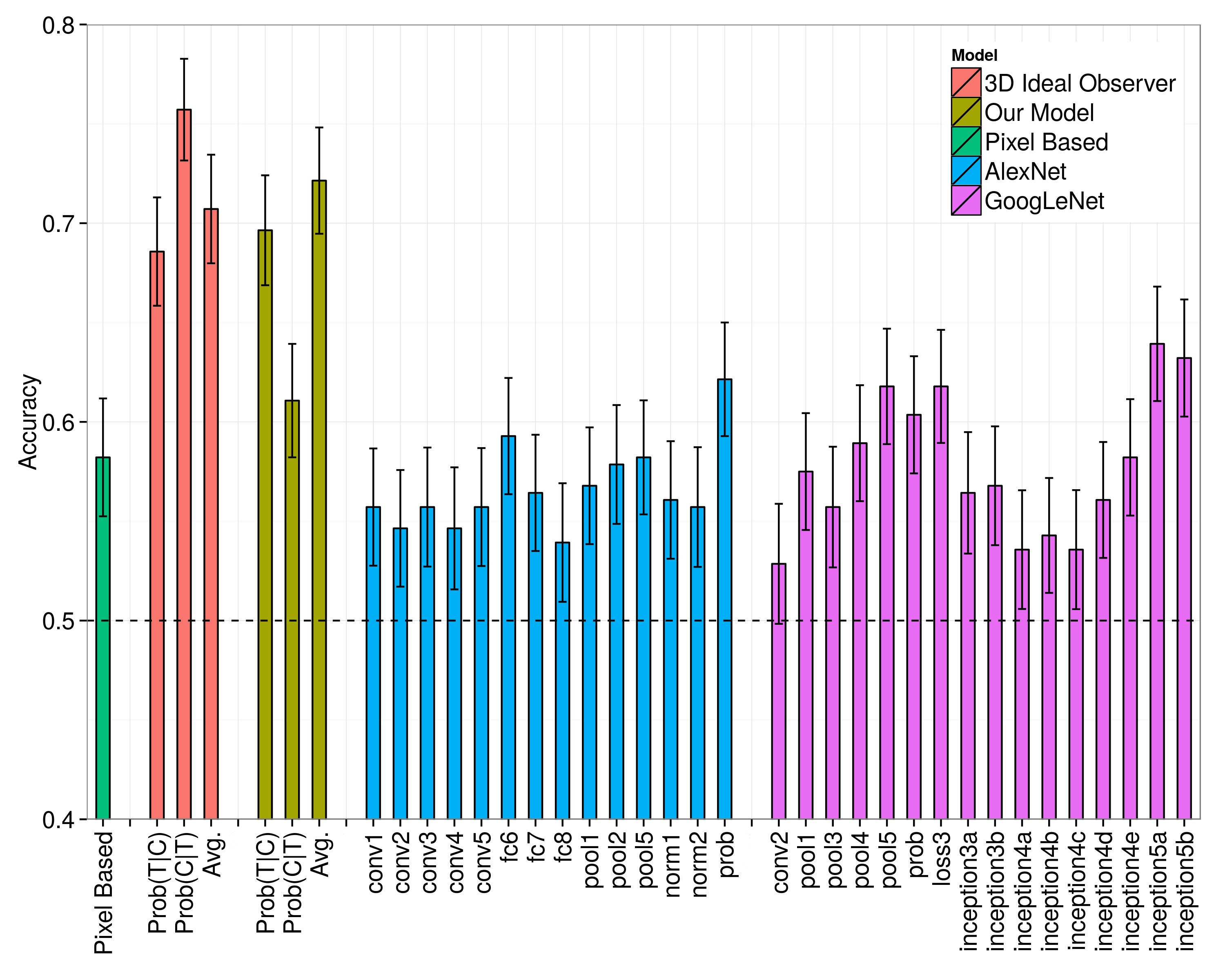## CogSci 2016 Submission Supplementary Materials

This page contains the supplementary materials for our submission to CogSci 2016 conference.

Erdogan G., Jacobs R. A. A 3D shape inference model captures human performance better than deep convolutional neural networks.

Code for our 3D shape inference model is available online at https://github.com/gokererdogan/Infer3DShape/releases/tag/cogsci16.

### Experimental Stimuli

In the paper, we only show some example stimuli. Below you can see the full set of 90 images used in the experiment. Variations cs: change part size, ap: add part, rp: remove part, mf: change docking face of part. d2 (depth 2) and d3 (depth 3) refer to the level at which the manipulation is applied.

## Results

### Samples

Here are some more examples of samples from our model.

### Model Comparison

In the paper, we only show the figure comparing model performances on all trials. Below is the figure showing model performances only on the high confidence trials.For completeness sake, here is the figure for all trials (Figure 4 in our paper).### Fitting CNN outputs to subject data

We assume that shape representations used by our subjects might be some linearly transformed version of the representations learned by CNNs. Subjects’ judgments in our experiment can be thought of as relative similarity constraints; for example, if subjects picked $$I_i$$ to be more similar to $$I_j$$ than $$I_k$$ is, this can be encoded as a constraint of the form $$s(I_i, I_j) > s(I_i, I_k)$$. Therefore, we need to learn a linear transformation that satisfies as many of these constraints as possible. Metric learning aims to learn a linear transformation $$G$$ of shape representations $R(I)$ such that the distances $$||G R(I_i) - G R(I_j)||$$ and $$G_M(I_i) - G_M(I_k)||$$ capture subjects’ judgments, i.e., satisfy the relative similarity constraints. This problem can be stated as an optimization problem that can be solved by iterative methods. In order to evaluate each model, we split 70\% of subjects’ similarity judgments into a training set and use the rest as our test set. We learn the linear transformation that maximizes the performance on training set and evaluate performance on the test. We repeat this procedure 50 times to get a performance estimate for each model. We try both diagonal and low-rank $$G$$ matrices with varying number of rank and report the best results. Tables below shows the performance on all trials and only high-confidence trials for pixel-based model, AlexNet and GoogLeNet. Metric learning seems to help only AlexNet; however, this increase in performance is not significant (p=0.18). Importantly, our model still outperforms all other models significantly (p=0.03 for comparison with AlexNet). If we focus on only high confidence trials, metric learning improves the performance of all models, albeit still not significantly (p>0.05 for all models). Again, our 3D shape inference model is significantly better than all other models (p=0.003 for comparison with AlexNet). These results show that, even if we fit the representations learned by these models to subject data, our model that uses 3D representations better accounts for subjects’ judgments.

Model Metric type Accuracy Best accuracy w/o metric learning
AlexNet (prob) low rank, r=20 0.660 0.621
GoogLeNet (inception5b) low rank, r= 20 0.633 0.639
Pixel-based low rank, r=10 0.566 0.582

Model Metric type Accuracy Best accuracy w/o metric learning
AlexNet (prob) low rank, r=5 0.752 0.733
GoogLeNet (inception5b) diagonal 0.715 0.683
Pixel-based low rank, r=10 0.698 0.616

### Subject Data and Model Predictions

Below we also provide detailed results for each model and our experimental data.